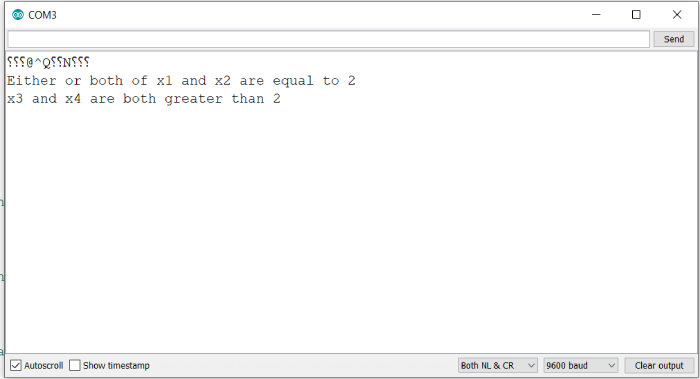# Logical AND and OR in Arduino

The logical AND is denoted by the && operator, while the logical OR is denoted by the || operator.

## Syntax

Expression1 && Expression2

OR

Expression1 || Expression2

Where expression1 and expression2 evaluate to Boolean values (true or false). The output of these statements is determined by the truth tables of logical AND and OR.

The truth table for AND is given below −

Expression1Expression2Output
TTT
FTF
TFF
FFF

As you can see, both the expressions have to be true for the AND statement to output true.

The truth table for OR is given below −

Expression1Expression2Output
TTT
FTT
TFT
FFF

As you can see, even if one of the expressions is true, the OR statement will output true.

## Example

The following example demonstrates the use of these operators −

void setup() {
// put your setup code here, to run once:
Serial.begin(9600);
Serial.println();

int x1 = 1;
int x2 = 2;
int x3 = 3;
int x4 = 4;

if((x1 > 2) && (x2 < 2)){
Serial.println("x1 is greater than 2 and x2 is less than 2");
}

if((x1 == 2) || (x2 == 2)){
Serial.println("Either or both of x1 and x2 are equal to 2");
}

if((x3 > 2) && (x4 > 2)){
Serial.println("x3 and x4 are both greater than 2");
}

if((x3 < 2) || (x4 < 2)){
Serial.println("Either or both of x3 and x4 are less than 2");
}
}

void loop() {
// put your main code here, to run repeatedly:
}

## Output

The Serial Monitor output is −• In the first case, both expressions were false, so the AND output was false, and nothing was printed.

• In the second case, one expression was true, so the OR expression was true, and the statement was printed.

• In the third case, both the expressions were true, so the AND output was true and the statement was printed.

• In the fourth case, both the expressions were false, so the OR output was false, and nothing was printed.

Updated on: 31-May-2021

10K+ Views